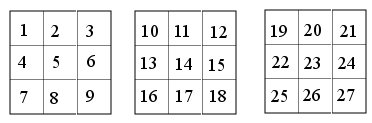# CAIA surprised me Part I

An AI researcher can always arrange his system so that it can find a solution that he already knows. To do that, he chooses a formulation well adapted to this solution, he includes all the methods necessary for this solution, and he indicates to avoid the roads that do not lead to the right direction. For this reason, it is always interesting to see a system finding a solution unknown by its author.

We have already seen how ALICE, developed by Jean-Louis Laurière, had immediately solved a problem proposed by professor Schutzenberger. I will now describe how CAIA has solved a problem better than I would have done.

Let us consider a kind of magic cube: the goal is to find a bijection F between the 27 small cubes that are in a 3x3x3 large cube, and the integers between 1 and 27. The sums of the planes containing nine cubes must have the same value VAL. There are 15 such planes: 3 horizontal, 6 vertical, and 6 diagonal. The small cubes are numbered in the following way, the first array is for the upper face, and the third one for the lower face, as shown below:The three constraints for the horizontal planes are:

F(1)+F(2)+F(3)+F(4)+F(5)+F(6)+F(7)+F(8)+F(9) = VAL

F(10)+F(11)+F(12)+F(13)+F(14)+F(15)+F(16)+F(17)+F(18)=VAL

F(19)+F(20)+F(21)+F(22)+F(23)+F(24)+F(25)+F(26)+F(27)=VAL

The six constraints for the vertical planes:

F(1)+F(2)+F(3)+F(10)+F(11)+F(12)+F(19)+F(20)+F(21)=VAL

F(4)+F(5)+F(6)+F(13)+F(14)+F(15)+F(22)+F(23)+F(24)=VAL

F(7)+F(8)+F(9)+F(16)+F(17)+F(18)+F(25)+F(26)+F(27)=VAL

F(1)+F(4)+F(7)+F(10)+F(13)+F(16)+F(19)+F(22)+F(25)=VAL

F(2)+F(5)+F(8)+F(11)+F(14)+F(17)+F(20)+F(23)+F(26)=VAL

F(3)+F(6)+F(9)+F(12)+F(15)+F(18)+F(21)+F(24)+F(27) = VAL

The last six constraints are for the diagonal planes:

F(1)+F(2)+F(3)+F(13)+F(14)+F(15)+F(25)+F(26)+F(27)=VAL

F(7)+F(8)+F(9)+F(13)+F(14)+F(15)+F(19)+F(20)+F(21)=VAL

F(1)+F(4)+F(7)+F(11)+F(14)+F(17)+F(21)+F(24)+F(27) = VAL

F(3)+F(6)+F(9)+F(11)+F(14)+F(17)+F(19)+F(22)+F(25)=VAL

F(1)+F(5)+F(9)+F(10)+F(14)+F(18)+F(19)+F(23)+F(27)=VAL

F(3)+F(5)+F(7)+F(12)+F(14)+F(16)+F(21)+F(23)+F(25)=VAL

In this old version of CAIA, I have indicated that the value of VAL was between 1 and 300. This was a very small aid; in the future versions, telling that VAL is positive will be enough.

I have already drawn attention to the importance of the problem formulation. To sum up, CAIA knows that it must find a bijection of 27 unknowns on the set of integers from 1 to 27, and the value of the positive unknown VAL, less than 300, such that the 15 preceding constraints are satisfied.

One could think that this is an easy problem, since it is under-constrained: there are 28 unknowns and only 15 constraints, plus the fact that one has a bijection. It has millions of solutions. However, the search tree is very large since every F has 27 possible values, and 300 for VAL. Moreover, each constraint contains 10 unknowns, and one must instantiate 9 of them to get a value for the tenth: many instantiations have to be made before one sees a contradiction. From this point of view, the problem is extremely difficult to solve: there are many solutions, but they are hidden in billions of possible instantiations for the unknowns.

We will see in the following posts how CAIA solved this problem, beginning with the discovery of its symmetries.